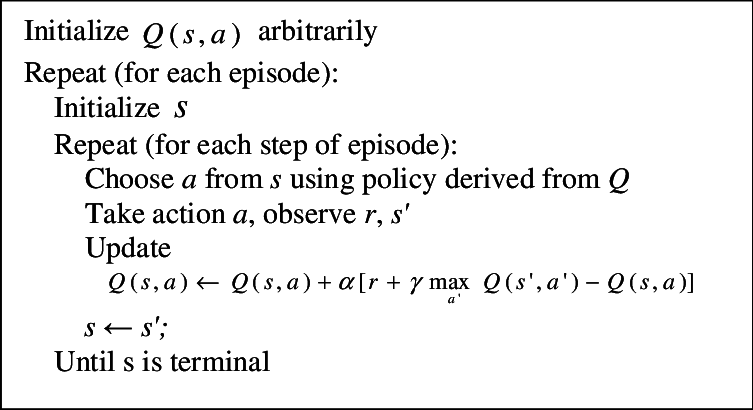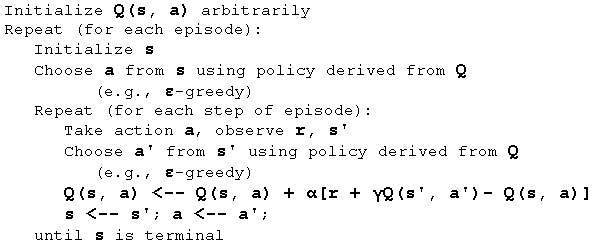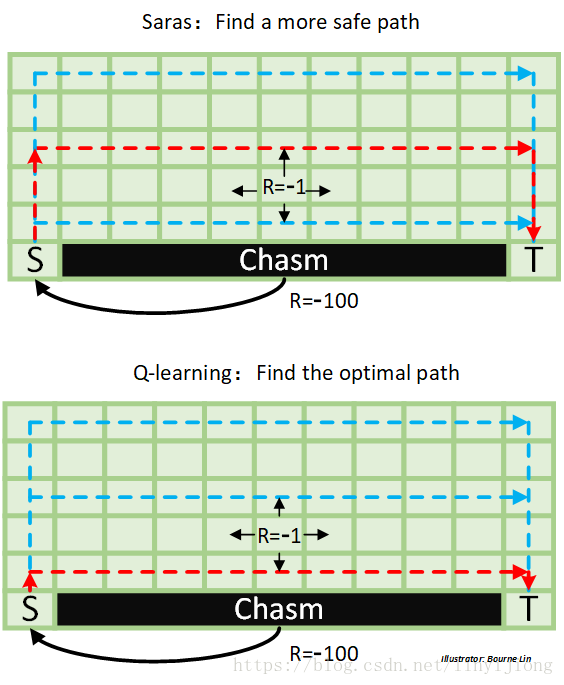# Qlearning和Sarsa的区别到底是什么？为什么说Qlearning勇敢而Sarsa胆小谨慎？

## 正文开始

Qlearning和Sarsa真的很像，如果已经有了其中一个代码，只需稍作修改就可以得到另一个。

## 说到底的区别

Qlearning:Sarsa:## 那么为啥Sarsa胆小呢## 总个破结

Qlearning

``````def learn(self, s, a, r, s_):
self.check_state_exist(s_)
q_predict = self.q_table.loc[s, a]
if s_ != 'terminal':
q_target = r + self.gamma * self.q_table.loc[s_, :].max()
else:
q_target = r  # next state is terminal
self.q_table.loc[s, a] += self.lr * (q_target - q_predict)  # update
``````

Sarsa

``````def learn(self, s, a, r, s_, a_):
self.check_state_exist(s_)
q_predict = self.q_table.loc[s, a]
if s_ != 'terminal':
q_target = r + self.gamma * self.q_table.loc[s_, a_]
else:
q_target = r  # next state is terminal
self.q_table.loc[s, a] += self.lr * (q_target - q_predict)
``````

THE END